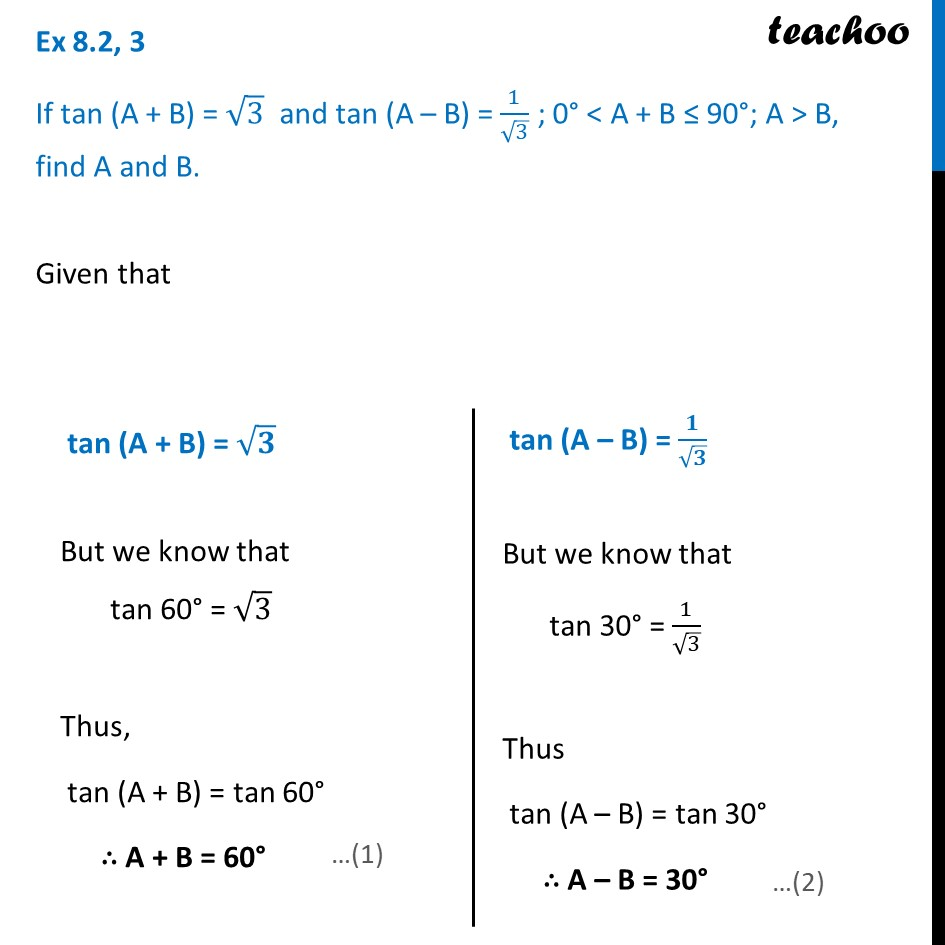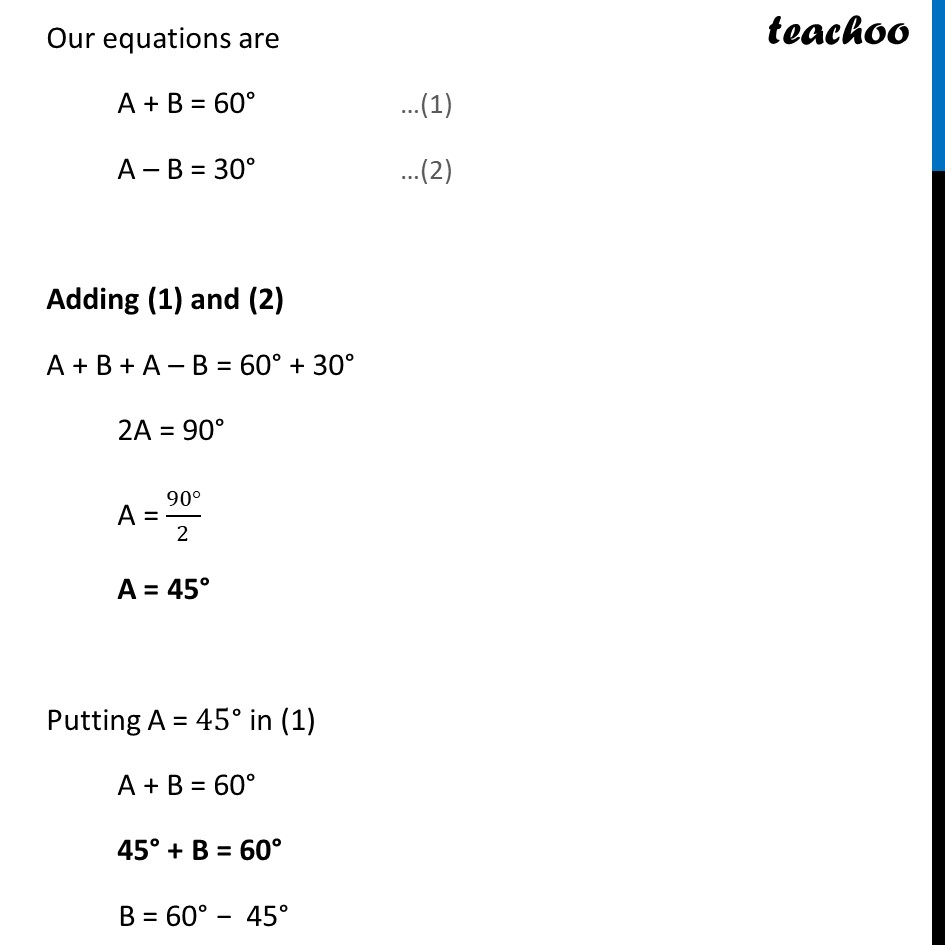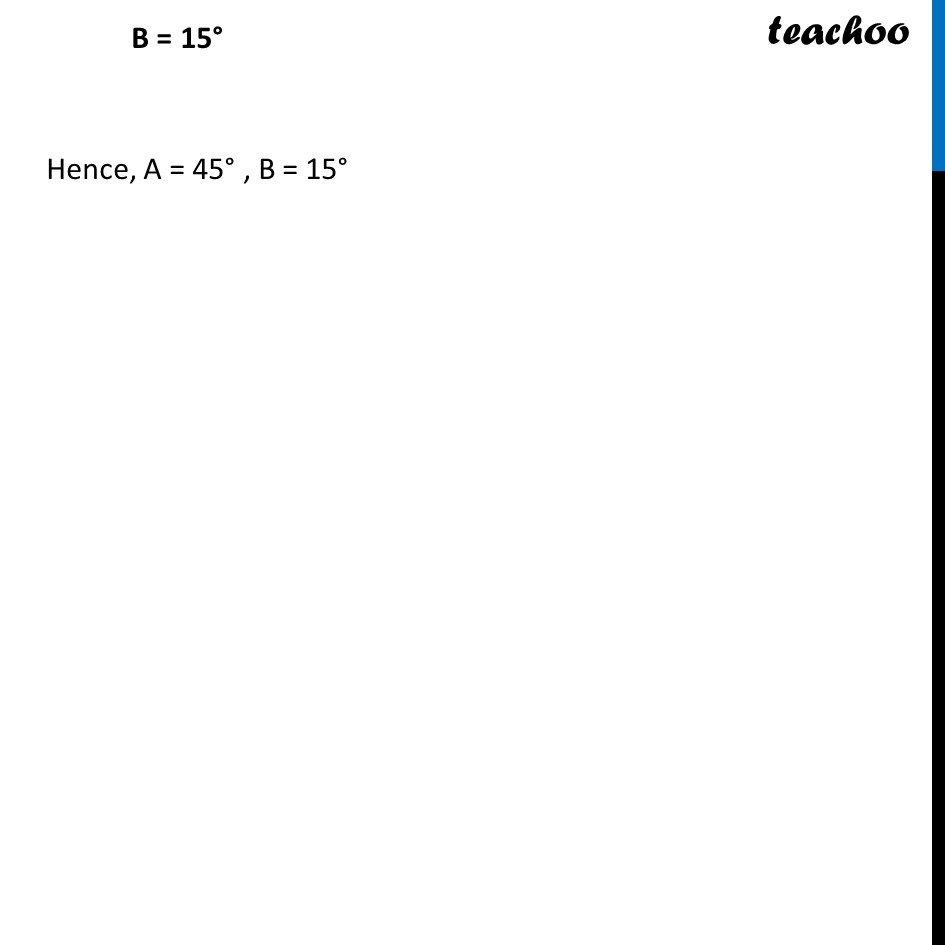Ex 8.2

Chapter 8 Class 10 Introduction to Trignometry
Serial order wiseLearn in your speed, with individual attention - Teachoo Maths 1-on-1 Class

### Transcript

Ex 8.2, 3 If tan (A + B) = √3 and tan (A – B) = 1/√3 ; 0° < A + B ≤ 90°; A > B, find A and B. Given that tan (A + B) = √𝟑 But we know that tan 60° = √3 Thus, tan (A + B) = tan 60° ∴ A + B = 60° tan (A – B) = 𝟏/√𝟑 But we know that tan 30° = 1/√3 Thus tan (A – B) = tan 30° ∴ A – B = 30° Our equations are A + B = 60° …(1) A – B = 30° …(2) Adding (1) and (2) A + B + A – B = 60° + 30° 2A = 90° A = (90°)/2 A = 45° Putting A = "45°" in (1) A + B = 60° 45° + B = 60° B = 60° − 45° B = 15° Hence, A = 45° , B = 15°